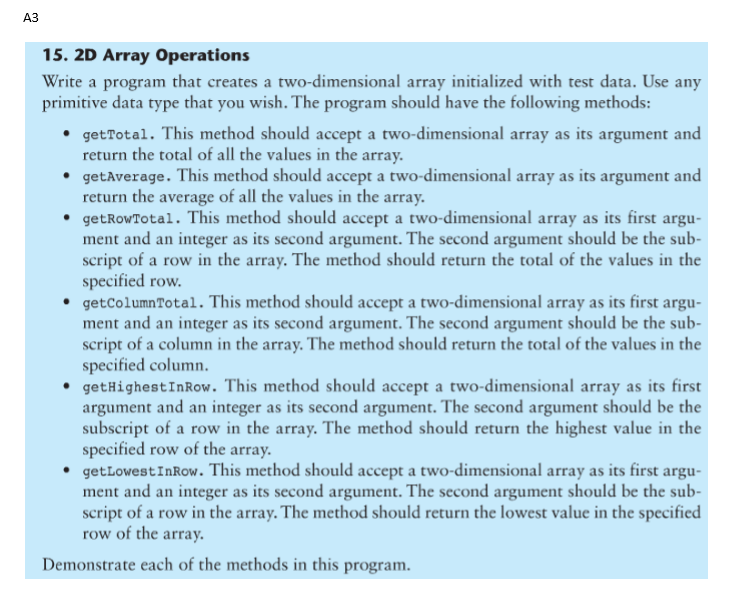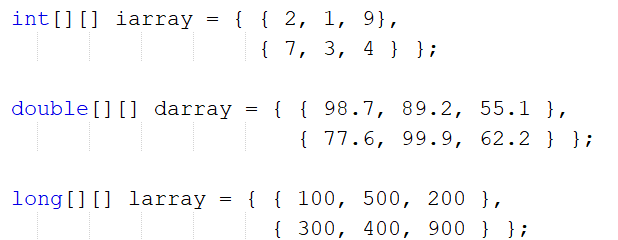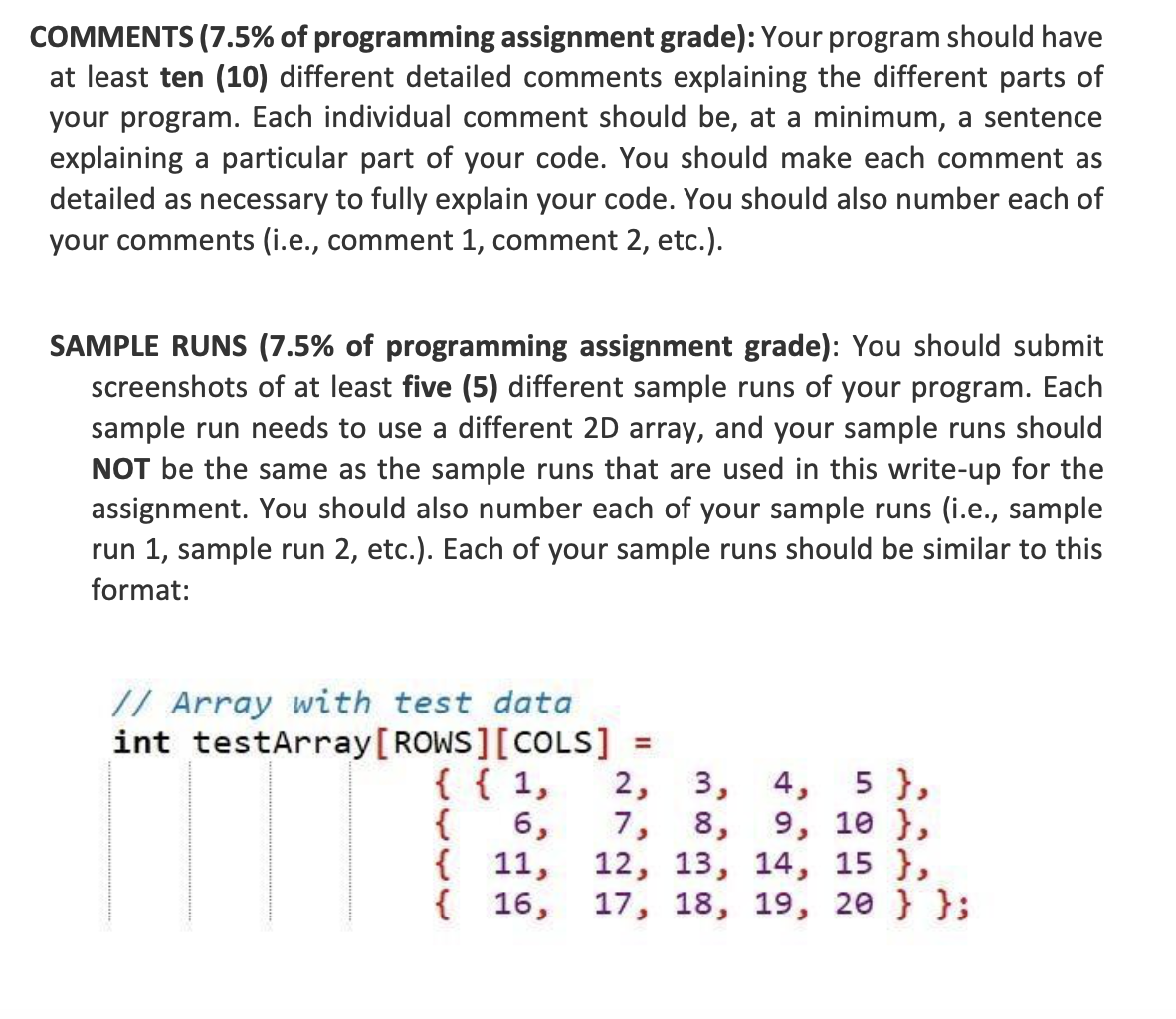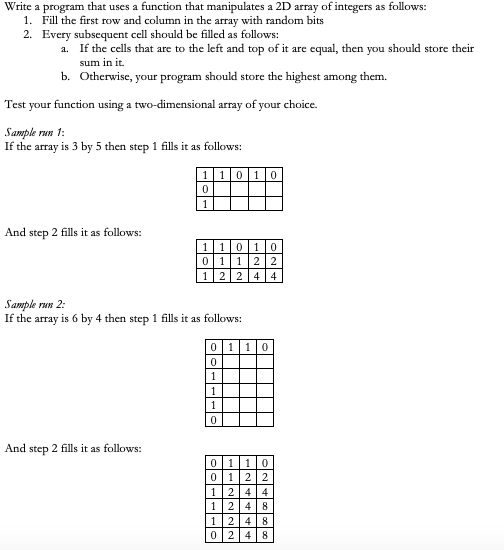Question

# 2D array operations

Write a program that creates a two-dimensional array initialized with test data. Use any primitive data type you wish. The program should have the followingmethods.
• getTotal - This method should accept a two-dimensional array as its argument and return the total of all the values in the array.
• getAverage - This method should accept a two-dimensional array as its argument and return the average of all the values in the array.
• getRowTotal. - This method should accept a two-dimensional array as its first argument and an integer as its second argument. The second argument should be thesubscript of a row in the array. The method should return the total of the values in the specified row.
• getColumnTotal - This method should accept a two-dimensional array as its First argument and an integer as its second argument. The second argument should be thesubscript of a column in the array. The method should return the total of the values in the specified column.
• getHighestInRow - This method should accept a two-dimensional array as its First argument and an integer as its second argument. The second argument should be thesubscript of a row in the array. The method should return the Highest values in the specified row in the array.
• getLowestInRow - This method should accept a two-dimensional array as its First argument and an integer as its second argument. The second argument should be thesubscript of a row in the array. The method should return the lowest values in the specified row in the array.

** please comment each method (mentioned above) so I know what I am looking at. I am pretty lost and need to learn this. Thank you
Document your code, with annotated code throughout. Think of what comments needed if you were to look at the program a year from now.
You can initialize the array's data in the declaration to save time. Create methods to operate on two types of arrays, which means you'll have twelve methods. Use aclass that performs the array operations, and a class that creates an instance of this class, for example:

ArrayOperations2D.java
ArrayOperations2DDemo.java

Here's some example output:

We need at least 10 more requests to produce the answer.

0 / 10 have requested this problem solution

The more requests, the faster the answer.

All students who have requested the answer will be notified once they are available.

#### Earn Coins

Coins can be redeemed for fabulous gifts.

Similar Homework Help Questions
• ### 2-Dimensional Array c++

Write a program that create a two-dimensional array initialized with test data. The program should have the following functions:getTotal - This function should accept two-dimensional array as its argument and return the total of all the values in the array.getAverage - This function should accept a two-dimensional array as its argument and return the average of values in the array.getRowTotal - This function should accept a two-dimensional array as its first argument and an integer as its second argument. The...

• ### Java Programming (use jGrasp if not thats fine) Write a Two classes one class that creates...

Java Programming (use jGrasp if not thats fine) Write a Two classes one class that creates a two-dimensional 2 rows by 3 columns double array. This class will have a separate method for the user to populate the array with data values. The other class that has the following methods:   getTotal. This method should accept a two-dimensional array as its argument and return the total of all the values in the array.   getAverage. This method should accept a two-dimensional array...

• ### Programming Challenge 12, Chapter 8; Starting Out with Java 4th Edition. Tony Gaddis.

Write a program that creates a two-dimensional array initialized with test data. Use any primitive data type that you wish. The program should have the followingmethods:-getTotal: This method should accept a two-dimensional array as its argument and return the total of all the values in the array.-getAverage: This method should accept a two-dimensional array as its argument and return the average of all the values in the array.getRowTotal: This method should accept a two-dimensional array as its first argument and...

• ### This is java, please follow my request and use netbeans. Thank you. A3 15. 2D Array...This is java, please follow my request and use netbeans. Thank you. A3 15. 2D Array Operations Write a program that creates a two-dimensional array initialized with test data. Use any primitive data type that you wish. The program should have the following methods: • getTotal. This method should accept a two-dimensional array as its argument and return the total of all the values in the array. .getAverage. This method should accept a two-dimensional array as its argument and return...

• ### Java Programming Assignment Write a class named 2DArrayOperations with the following static methods: getTotal . This...Java Programming Assignment Write a class named 2DArrayOperations with the following static methods: getTotal . This method should accept a two-dimensional array as its argument and return the total of all the values in the array. Write overloaded versions of this method that work with int , double , and long arrays. (A) getAverage . This method should accept a two-dimensional array as its argument and return the average of all the values in the array. Write overloaded versions of...

• ### Please solve only if you know how to do it. Write the code using C++ (not...Please solve only if you know how to do it. Write the code using C++ (not Python or Java). Show and explain everything neatly. COMMENTS (7.5% of programming assignment grade): Your program should have at least ten (10) different detailed comments explaining the different parts of your program. Each individual comment should be, at a minimum, a sentence explaining a particular part of your code. You should make each comment as detailed as necessary to fully explain your code. You...

• ### - Write a program that performs several operations on an array of positive ints. The program...

- Write a program that performs several operations on an array of positive ints. The program should prompt the user for the size of the array (validate the size) and dynamically allocate it. Allow the user to enter the values, do not accept negative values in the array. - Your program should then define the functions described below, call them in order, and display the results of each operation: a) reverse: This function accepts a pointer to an int array...

• ### . In the method main, prompt the user for a non-negative integer and store it in...

. In the method main, prompt the user for a non-negative integer and store it in an int variable num (Data validation is not required for the lab). Create an int array whose size equals num+10. Initialize the array with random integers between 0 and 50 (including 0 and excluding 50). Hint: See Topic 4 Decision Structures and File IO slides page 42 for how to generate a random integer between 0 (inclusive) and bound (exclusive) by using bound in...

• ### In C++ for 2D Array: Write a program that uses a function that manipulates a 2D...In C++ for 2D Array: Write a program that uses a function that manipulates a 2D array of integers as follows: 1. Fill the first row and column in the array with random bits 2. Every subsequent cell should be filled as follows: 1. If the cells that are to the left and top of it are equal, then you should store their sum in it b. Otherwise, your program should store the highest among them. Test your function using...

• ### Using Java: 1. Recursive Multiplication Write a recursive function that accepts two arguments into the parameters...

Using Java: 1. Recursive Multiplication Write a recursive function that accepts two arguments into the parameters x and y. The function should return the value of x times y. Remember, multiplication can be performed as repeated addition as follows: 5×6=6+6+6+6+6 2. Recursive findings Write a recursive boolean method named reFinding. The method should search an array for a specified value, and return true if the value is found in the array, or false if the value is not found in...